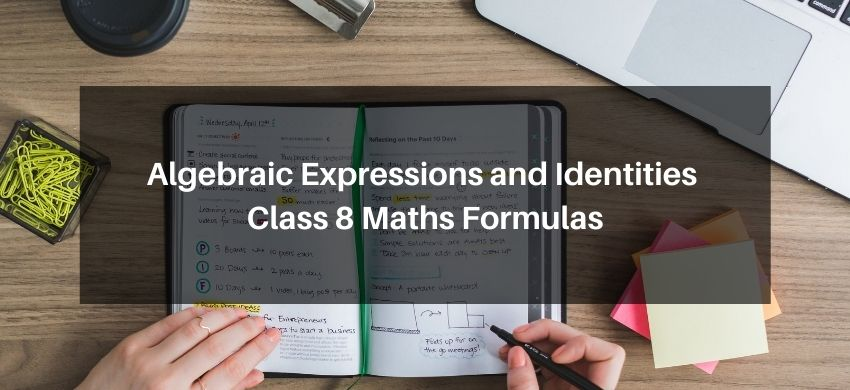Most Affordable JEE | NEET | 8,9,10 Preparation by Kota's Top IITian Doctor Faculties

# Algebraic Expressions and Identities Class 8 Maths FormulasHey, students are you looking for Algebraic Expressions and Identities Class 8 Maths Formulas? If yes. Then you are at the right place. In this post, I have listed all the formulas of Algebraic Expressions and Identities class 8 that you can use to learn and understand the concepts easily.

If you want to improve your class 8 Math, Algebraic Expressions and Identities concepts, then it is super important for you to learn and understand all the formulas.

By using these formulas you will learn about Algebraic Expressions and Identities.

With the help of these formulas, you can revise the entire chapter easily.

## Algebraic Expressions and Identities Class 8 Maths Formulas

• $(a+b)^{2}=a^{2}+2 a b+b^{2}$
• $(a-b)^{2}=a^{2}-2 a b+b^{2}$
• $(a+b)(a-b)=a^{2}-b^{2}$
• $(x+a)(x+b)=x^{2}+(a+b) x+a b$
• $(x+a)(x-b)=x^{2}+(a-b) x-a b$
• $(x-a)(x+b)=x^{2}-(a-b) x-a b$
• $(a+b)^{3}=a^{3}+b^{3}+3 a b(a+b)$
• $(a-b)^{3}=a^{3}-b^{3}-3 a b(a-b)$
• $a^{3}-b^{3}=(a-b)\left(a^{2}+a b+b^{2}\right)$
• $a^{3}+b^{3}=(a+b)\left(a^{2}-a b+b^{2}\right)$
• $x(a+b)=x a+x b$
• $x(a-b)=x a-x b$
• $(x-a)(x-b)=x^{2}-(a+b) x+a b$
• $(x-a)(x+b)=x^{2}+(b-a) x-a b$
• $(x+a)(x-b)=x^{2}+(a-b) x-a b$
• $(x+a)(x+b)=x^{2}+(a+b) x+a b$
• $(x+y+z)^{2}=x^{2}+y^{2}+z^{2}+2 x y+2 y z+2 z x$
• $(x-y-z)^{2}=x^{2}+y^{2}+z^{2}-2 x y+2 y z-2 z x$
• If $x$ is a variable and $m, n$ are positive integers, then $\left(x^{m} \times x^{n}\right)=x^{(m+n)}$ Thus, $x^{2} \times x^{4}=x^{(2+4)}=x^{6}$
• If $x$ is a variable and $m, n$ are positive integers such that $m>n$, then $\left(x^{m} \div x^{n}\right)=x^{m-n} \cdot$ Thus, $x^{9} \div x^{4}=x^{9-4}=x^{5}$
• Product of two monomials $=$ (Product of their coefficients) $\times$ (Product of their variables)
• Division of two monomials $=$ (Division of their coefficients) $\times$ (Division of their variables)

NCERT Class 8 Maths Book in PDF

NCERT Class 8 Science Book in PDF

NCERT Class 8 Science Exemplar in PDF

NCERT Class 8 Maths Exemplar in PDF

If you have any Confusion related to Algebraic Expressions and Identities Class 8 Maths Formulas, then feel free to ask in the comments section down below.

To watch Free Learning Videos on Class 8 Maths by Kota’s top Faculties Install the eSaral App